##### Actions

A problem attributed, to J.H.C. Whitehead, which asks for a characterization of Abelian groupsthat satisfy the homological condition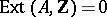, whereis the group of integers under addition (cf. also Homology). A group which satisfies this condition is called a Whitehead group. An equivalent characterization is:is a Whitehead group if and only if for every surjective homomorphismonto, if the kernel ofis isomorphic to, then the kernel is a direct summand of the domain of. A sufficient condition forto be a Whitehead group is thatis free (see Free Abelian group). This condition has been proved to be necessary ifis countable. Since a subgroup of a Whitehead group is again a Whitehead group, this means that every Whitehead group is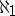-free, that is, every countable subgroup is free. Before 1973 only partial results were obtained for uncountable groups: Whitehead groups were proved to be separable and slender, and under the assumption of the continuum hypothesis (), they were proved to be strongly-free, that is, every countable subset is contained in a countable free subgroup which is a direct summand of countable subgroups containing it (see [a3] for results prior to 1973).
In 1973, S. Shelah [a5] proved that it is undecidable under the axioms of Zermelo–Frankel set theory (cf. also Set theory), ZFC, whether every Whitehead group of cardinalityis free. Specifically, he proved that this is true assuming the Gödel constructibility axiom,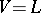(see Gödel constructive set), but it is false assuming Martin's axiom (cf. Suslin hypothesis) and the negation of the continuum hypothesis (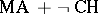). Later he proved thatimplies that every Whitehead group, of arbitrary cardinality, is free [a6]. He also proved that the problem is undecidable even if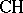is assumed [a7] and that it is consistent with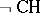that there are Whitehead groups of cardinalitythat are not strongly-free [a8].